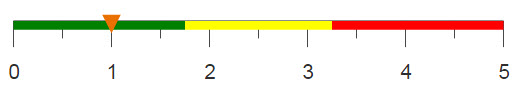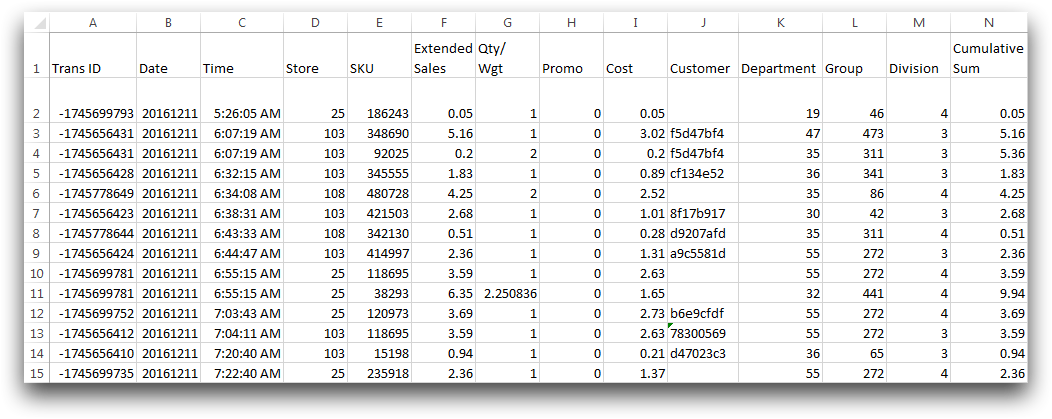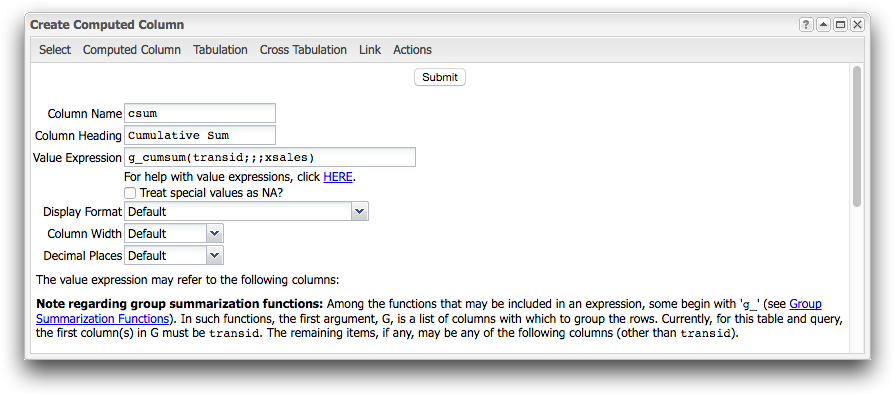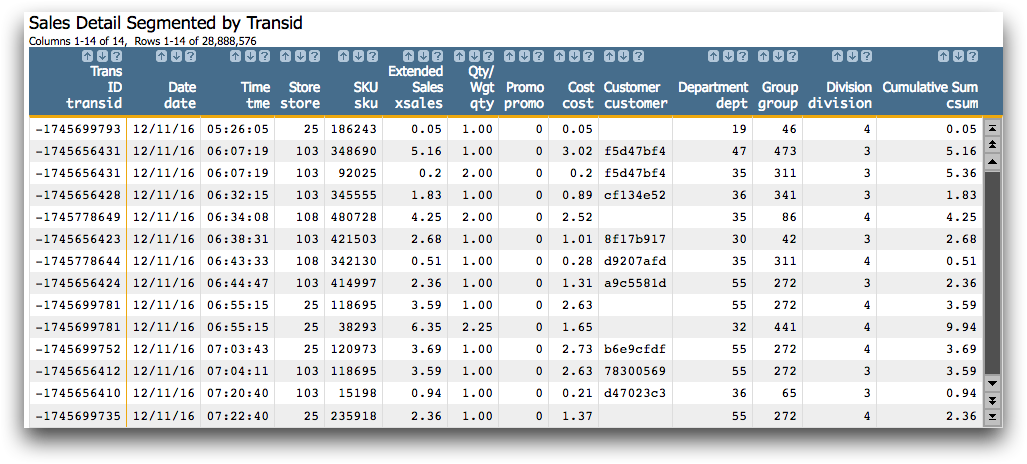# Computing multiple cumulative sums

Cumulative sums can be useful to distinguish individual sums of several different groups.

## Difficulty## Objective

You have a table consisting of sales transactions and you would like to compute the cumulative sum for each customer's purchase. You know how to do this in Excel using the SUMIF function, and you would like to perform the same operation using 1010data.

## Excel solution

In Excel, you can compute a cumulative sum for each desired group using some manipulation of the SUMIF function. The below formula will produce a cumulative sum for each customer's purchase.

`=SUMIF(A\$2:A2,A2,F\$2:F2)`

And your resulting table should look like the following image.A simple cumulative sum can be calculated using the SUM function, and using the Subtotal tool, you can obtain a sales subtotal for each transaction. However, in order to obtain a cumulative subtotal for each transaction, you need to manipulate the SUMIF function to compute an individual cumulative sum for each unique transaction number. 1010data's cumulative sum g_function, g_cumsum(G;S;O;X), allows you to calculate each transaction cumulative sum with one simple expression.

Additionally, with Excel you can only compute the cumulative sums for a subset of the transactions because the sales detail table in its entirety is too large for Excel. With 1010data, you can perform these operations on the entire table at a much faster speed.

## 1010data GUI solution

From the 1010data menu bar click Columns > Create Computed Column to open the dialog shown below.In the text box that corresponds to Value Expression, enter the g_cumsum(G;S;O;X) function. The note section within the dialog relates that customer and transid must be used for the G argument. The S and O arguments are left blank and xsales is used for the X argument. Upon clicking Submit, the Sales Detail table will look like the worksheet seen below.## 1010data Macro Language solution

The same results can be obtained by entering the following macro code into the Edit Actions dialog.

```<base table="pub.doc.retail.altseg.sales_detail_transid"/>
<willbe name="csum" label="Cumulative Sum" value="g_cumsum(transid;;;xsales)"/>```